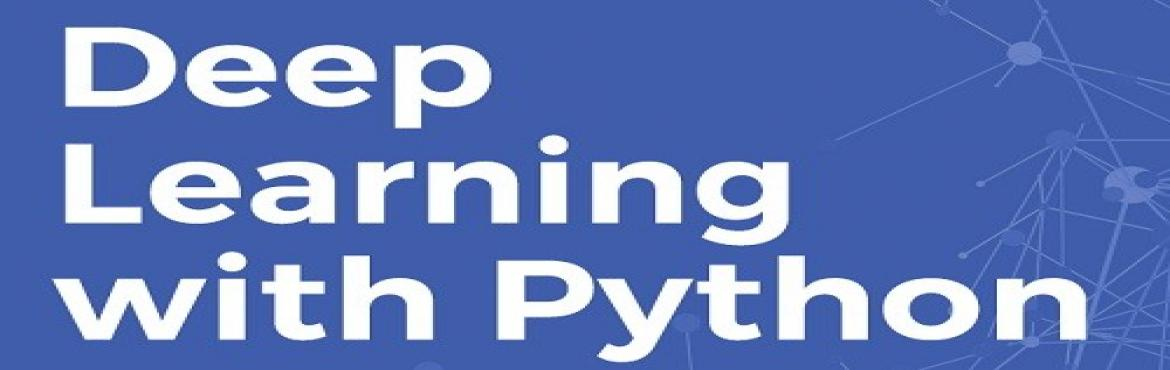# Deep Learning with Python

• ## Online & Offline

Sale Date Ended

INR 12000
Sold Out

#### Invite friends

Page Views : 22

Inspired by algorithms of Artificial Neural networks, this course will take you to the deeper neural network world. You will be acquainted with better estimation techniques of data science. Participants must have knowledge of Python.

## OBJECTIVE

Introductory Terms

• Data and Data Science.
• Big Data.
• Why Big Data.
• Math and Data Science.
• Introduction to Statistics.
• What is learning?
• Different type of learning.
• Introduction to Data mining, machine learning.
• Introduction to artificial intelligence.
• What is a model?
• Mathematical models.

NumPy Refresher :

• Introduction to NumPy.
• Ndarray.
• Array creation
• Matrix
• addition, subtraction, multiplication on Array
• Matrix multiplication.

MatPlotlib Refresher :

• Pyplot as submodule.
• Scatterplot
• lineplot
• histogram
• PiChart
• Bar Chart

TensorFlow Introduction :

• TensorFlow History.
• Installing TensorFlow.
• Introduction to Jupyter.
• TensorFlow with Jupyter.
• Introduction to tensor in context of tensor flow.
• TensorFlow Data types
• Computation and Dataflow graph
• Concept of session.
• Constant
• Placeholder
• Variables.

Mathematical operations in TensorFlow :

• Multiplication
• Summation
• Maximum
• Minimum
• Complex number operations.
• Some more mathematical functions.

Matrix operation and Linear algebra in TensorFlow :

• Matrix summation and Substraction.
• Matrix Transpose.
• Determinant of Matrix.
• Matrix multiplication.
• Inverse matrix.

Linear regression :

• Introduction to linear regression.
• Simple linear regression.
• Parameter estimations.
• Simple linear regression with TensorFlow.
• Evaluating our model.

Logistic Regression :

• Logistic Regression Introduction.
• Parameter estimation.
• With TensorFlow.
• Model Evaluation.

Clustering :

• Introduction to Clustering
• Kmeans
• Kmeans with TensorFlow
• Optimizing Kmeans
• Market Segmentation.

Deep Learning :

• Introduction
• Use cases
• Why I use deep learning ?

Introduction to Neural Network :

• Biological Neuron an Introduction.
• Component of biological Neuron.
• Artificial Neuron.
• Working of artificial neuron.
• Activation function
• Sigmoid function.
• Linear
• ReLU
• Tanh
• Concept of feed forward.
• AND, OR and NOT
• Perceptron.
• Perceptron learning algorithm.
• Implementing Perceptron in TensorFlow.

Multilayer perceptron :

• Backpropgation algorithm.
• MLP with TensorFlow.
• Classifying our data.

Convolutional  Neural networks (CNN) :

• Convolutional Neural networks Introduction.
• Convolutional Layer.
• Pooling Layer .
• Connecting fully.
• Image classification and Convolutional Networks.
• TensorFlow and CNN
• Image Classification with TensorFlow.
• Model evaluation.

Recurrent Neural network (RNN) :

• Introduction
• Back Propagation through time (BPTT)
• Need of Memory.
• Long Short Term memory (LSTM).
• Bi-Directional RNN
• Implementing RNN with TensorFlow.
• Time Series and RNN
• Sequence prediction with RNN.